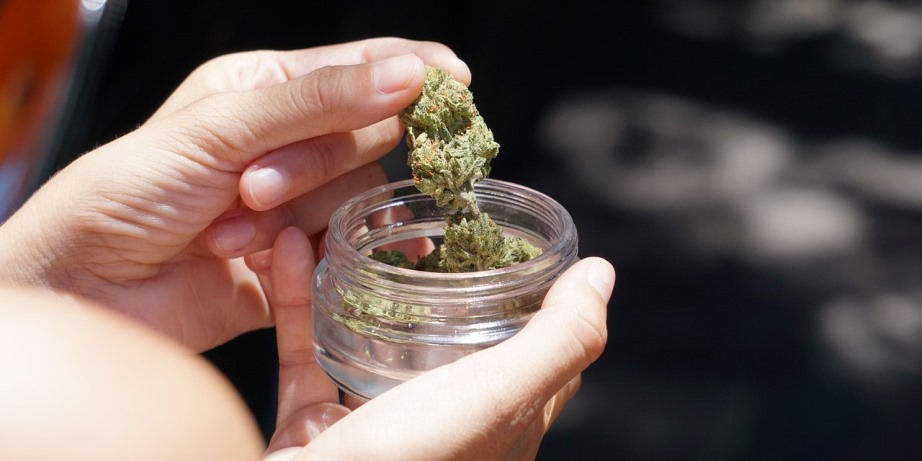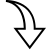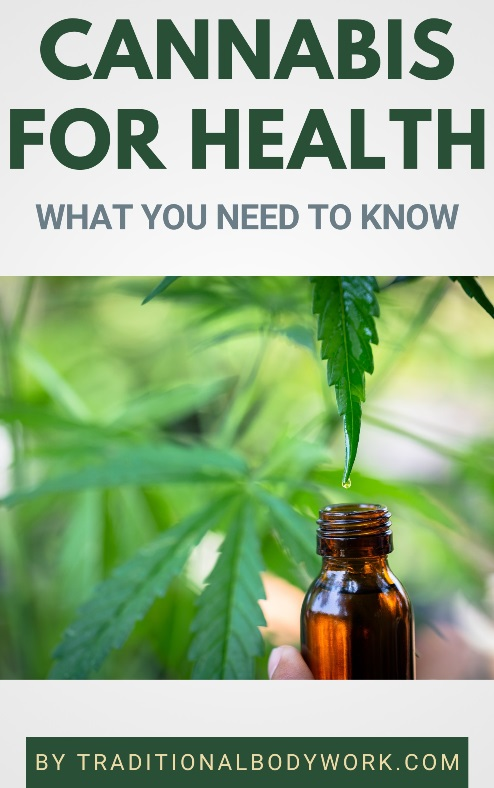# THC and CBD Potencies | Mg to Percentages and Vice Versa

Published: Dec 11, 2022When it comes to Cannabis, you will read and hear a lot about the 0.2% or 0.3% THC thresholds or potencies, for instance in order to know if a Cannabis plant or product is rather Hemp or Marijuana.

When you buy Cannabis products you will find labels on them that, for instance, state 5 mg/g THC or 100 mg/g CBD. In fact, as a general rule, Cannabis product labels will display THC (Tetrahydrocannabinol) and/or CBD (Cannabidiol) potencies in mg/g, or you will see the total amount of active milligrams in the product.eBookThe abbreviation mg stands for milligram and g stands for gram (1 gram = 1000 milligram). In the case of (bottled) oils, you will also see ml (milliliter). 1 liter = 1000 milliliter. Keep in mind that 1 milliliter (1ml) equals 1 gram.

Now, to know CBD or THC percentages of mg/g potencies you simply divide by 10. That means, in the case of the examples given above, that 5 mg/g THC is 0.5% THC and 100 mg/g CBD is 10% CBD.

This also works the other way around: 0.5% THC would mean 0.5% x 10 = 5 mg/g and 10% CBD would mean 10% x 10 = 100 mg/g.

If a product simply states the total amount of CBD and/or THC milligrams, you will need to know the total weight of the product to calculate. So, if it’s stated that the product contains 500 mg CBD and the bottle contains a total of 30 ml (milliliters) of oil, you will first need to convert ml (milliliters) to mg (milligram).

That is, 30 ml equals 30 g equals 30,000 mg. It means that this product contains 500 mg CBD for a total amount of 30,000 mg oil = 16.66 mg CBD per 1,000 mg (=1g), which would be 16.66 mg/g and calculates to a 1.6% CBD potency (16.66 divided by 10).

Mind also that 1 drop from a dropper (or pipet) typically contains 0.05 ml fluid (0.05 g = 50 mg fluid). Taking our example above it would mean that 1 drop from the bottle would contain 16.66 mg x 0.05 = 0.83 mg CBD.

Related Articles